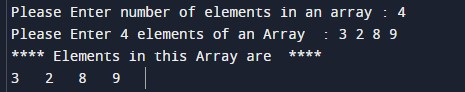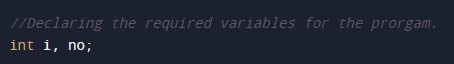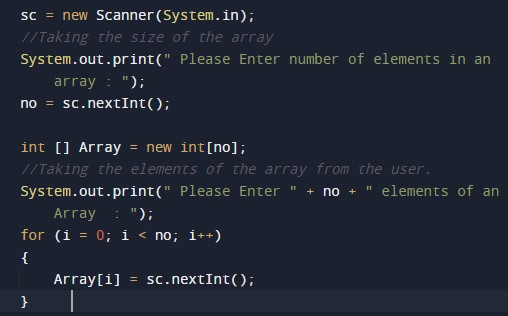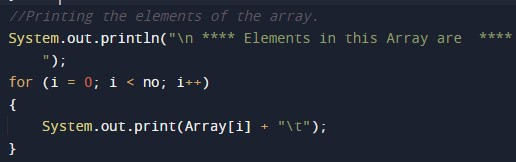# Java Program to print elements of an array

In this tutorial you will learn about the Java Program to print elements of an array and its application with practical example.

## Java Program to print elements of an array

In this tutorial, we will learn to create a Java program that will Print Elements in an Array using Java programming.

## Prerequisites

Before starting with this tutorial, we assume that you are the best aware of the following Java programming topics:

• Operators in Java Programming.
• Basic Input and Output function in Java Programming.
• Basic Java programming.
• For loop in Java programming.
• Arithmetic operations in Java Programming.

## What is an array?

An array in a programming language is a collection of a similar set of entities. It means one variable can store multiple values in memory with different indices.

## Printing the Elements in an Array.

In this program, First, we will first take the size of the array from the user. Then we will take the elements of the array from the user. After that, we will use a for loop to print the elements of the array.

With the help of this program, we can be print the elements of the array.

## Output:-In the above program, we have first initialized the required variable.• no = it will hold the integer value.
• i = it will hold the integer value.

Taking the size of the array and the elements.Printing output elements of the array.In this tutorial we have learn about the Java Program to print elements of an array and its application with practical example. I hope you will like this tutorial.